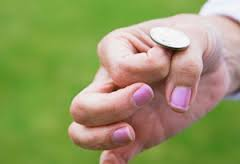# Eventually tossed a coin many times. Part-I

Probability Level 3A fair coin is tossed 10 times. If the probability of getting exactly six heads is $\dfrac{a}{b}$, where $a$ and $b$ are coprime positive integers, find the value $a+b$.

×